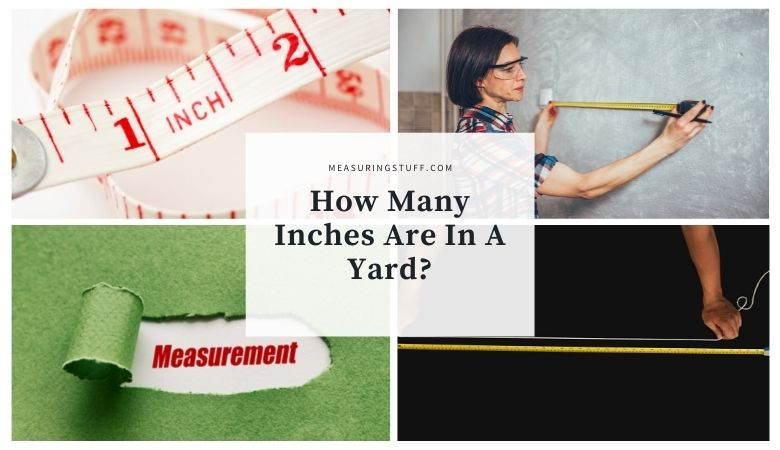# How Many Inches In A Yard? – YD To Inches Conversion Table

Converting the measurement of yards into inches can be done by using a simple formula.

1 Yard equals 36 inches. To convert yards into inches use the following formula:

Yards x 36 = inches

If you want to convert inches into yards, you need to divide by 36.

Inches / 36 = Yards

If you prefer to not do the math, you can use the yards to inches conversion table below.

## Inches Measurement

Inch or inches is a length unit of measurement. It is used in the USA as a customary and Imperial unit of length.

Many people will abbreviate the word inch as in. For example, 1 inch may be written as 1 in. It can also be denoted by using the double prime symbol , for example, 1 inch can be written as 1″.

1 inch is also equal to 1⁄36 yard or 1⁄12 foot.

## Yards Measurement

A yard is also a length unit of measurement used by both the British imperial and US customary systems of measurement.

1 yard is equal to 3 feet or 36 inches. An international yard is equal to 0.9144 inches.

The term yard can be abbreviated with the letters yd, for example 1 yard can be written as 1 yd.

## Yards To Inches Conversion Calculator

Use the following calculator to easily convert yards into inches.

For our complete section of unit conversions including tables and calculators click the link here.